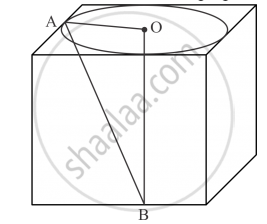Share

# Find the Volume of the Largest Right Circular Cone that Can Be Cut Out of a Cube Where Edge is 9cm_____? - Mathematics

Course

#### Question

Find the volume of the largest right circular cone that can be cut out of a cube where edgeis 9cm?

#### Solution

We have the following figureThe length of each side of the cube is 9 cm. We have to find the volume of the largest right circular cone contained in the cube.

The diameter of the base circle is same as the length of the side of the cube. Thus, the diameter of the base circle of the right circular cone is 9 cm. Therefore, the radius of the base of the right circular cone is r = 4.5cm.

From the right angled triangle ΔAOB we have

= 9 cm

Therefore, the volume of the solid right circular cone is

V = 1/3 pir^2h

= 1/3 xx 22/7 xx (4.5)^2 xx 9

 = 190.93

Hence largest volume of cone is  = 190.93cm^3

Is there an error in this question or solution?

#### APPEARS IN

RD Sharma Solution for Class 10 Maths (2018 (Latest))
Chapter 14: Surface Areas and Volumes
Ex. 14.1 | Q: 33 | Page no. 29
Solution Find the Volume of the Largest Right Circular Cone that Can Be Cut Out of a Cube Where Edge is 9cm_____? Concept: Volume of a Combination of Solids.
S